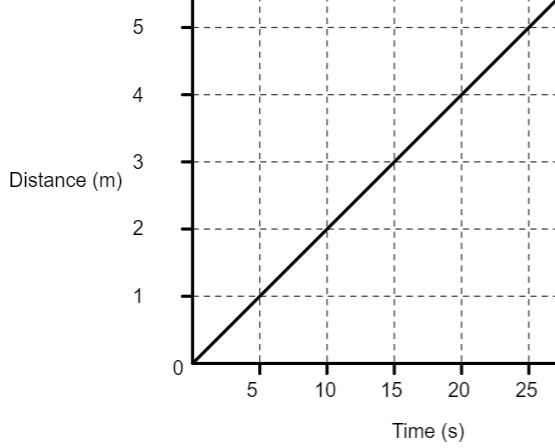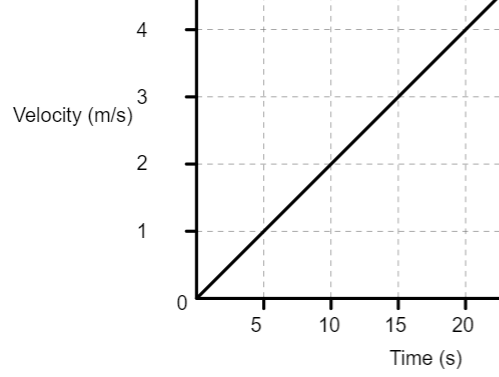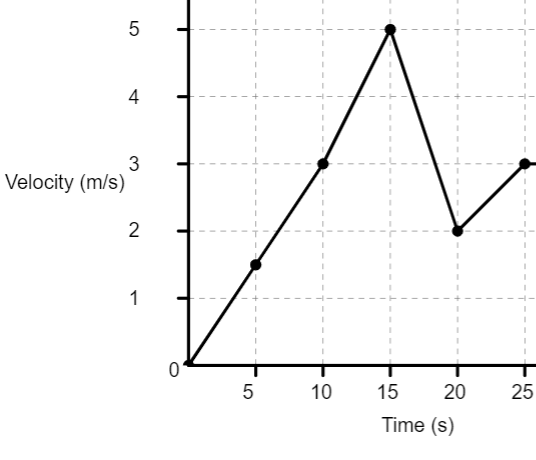Edumarz# Motion

Graphical representation of motion

Tanusri Gururaj, Academic content writer of Physics at Edumarz

Distance-time graph:

• Distance is along the y-axis and, time is along the x-axis.

• A distance-time graph is used to calculate the speed of the object. The slope of the graph gives the speed.

• The distance-time graph for an object moving at uniform speed is shown below-To calculate speed from the above graph, take two points A and, B on the line. Let us assume that point A is (10, 2) and point B is (25, 5).

Since the slope of the graph gives the speed,

Slope = speed = vertical difference/horizontal difference.

Speed = ((5-2)/(25 – 10)) m/s

Speed = 3/15 = 1/5 = 0.2 m/s

Similarly, we can calculate the velocity of an object if it is a displacement-time graph where displacement is along the y-axis and, time is along the x-axis.

Velocity-time graph:

• Velocity is along the y-axis and, time is along the x-axis.

• The area under the velocity-time graph represents the displacement as,

Displacement = Velocity x Time

• The slope of this graph gives the acceleration as acceleration is the rate of change of velocity.

• If the graph is parallel to the time axis it means that the velocity of the body is constant and, hence, the acceleration will be zero.

• The Velocity-time graph for an object moving with uniform acceleration is shown below.• The Velocity-time graph for an object moving with non-uniform acceleration is shown below.## AMU, a top-notch opportunity## AIMS Test for Management## BANARAS HINDU UNIVERSITY